## Recap

Last time, we used a set of arrows and vertices to represent a sequence of notes:

 such as the 'Cantus 1' line found in the first section of C T Padbrué's Synphonia in nuptias (for the marriage betwixt Steyn & van Napels) Your browser does not support the `audio` element. If the player doesn't work, there's some ogg vorbis over here.as a graph: reducible (eliminating uni-cycles and bi-cycles) to: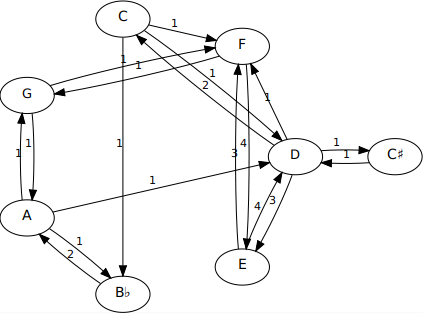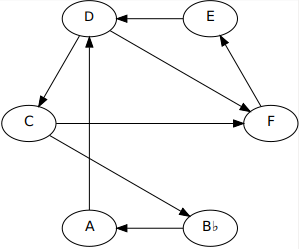whereupon we recovered the following three 'distillations' of the three lines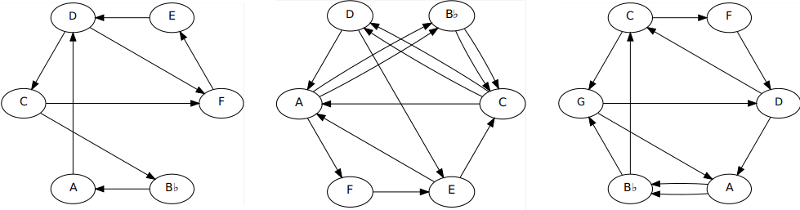Your browser does not support the `audio` element. We concocted a couple of examples using the reduced lines (as before, if the player doesn't work, there's some mp3 over here).

These directed graphs are known as quivers, consisting as they do of bunches of arrows, often many together (all facing the same direction) between vertex pairs since we've eliminated arrows which cancel each other out (i.e. all bi-cycles).

We wondered how many paths (if any) - consuming all arrows, exactly once - could be used to traverse each of these three graphs. It turns out (via nothing more sophisticated than brute force exhaustive searching) that for these three distillations there are (3, 130, 55) such paths. We show the three for cantus 1 and three typical ones for the other lines:

Cantus 1
(8 arrows)
Cantus 2
(13 arrows)
Bassus
(11 arrows)
C F E D C B♭ A D F
C B♭ A D C F E D F
C B♭ A D F E D C F
C D E C D A F E A B♭ C A B♭ C
D E C D A F E A B♭ C A B♭ C D
A F E A B♭ C A B♭ C D E C D A
G D C F D A B♭ C G A B♭ G
B♭ G D C G A B♭ C F D A B♭
D A B♭ C F D C G A B♭ G D

## Mutation Primer

The following describes an operation which - as someone said back in the 1990s when it was first being investigated - is elementary but complex.

We consider the idea of a quiver mutation as applied to these reduced melodic lines. It's necessary to perform these reductions before we mutate since the rules of mutation require it. The mutation is performed - reversibly - on a vertex of the quiver. In our case the vertex represents a musical pitch, but we don't change that pitch. Instead we alter its connections to its preceding and succeeding pitches by swapping their places and completing a circuit. Basically, the rules are - at the selected vertex:

1. Reverse all arrows entering and leaving the mutating vertex, M.
2. For every pair of vertices (P, S) now entering and exiting M (a 2-path from P(redecessor) to S(uccessor) via M), 'complete the circuit' PMS by adding a new arrow from S to P.
3. Maintain the 'no bi-cycle' constraint on the quiver by removing any newly introduced (by circuit completion) oppositely-directed arrow pairs.

We'll try to maintain a certain consistency of terminology here in distinguishing

• an arrow - a single directed edge (with one 'arrowhead') from one vertex to another, different one (no uni-cycles)
• a path - a trip from one vertex to another via arrows
Thus all arrows are paths (perforce of length 1) but not all paths are arrows. A path may start and finish at the same vertex - this is a cycle, or circuit. Thus all circuits are paths but not all paths are circuits. Note that quiver rules eliminate (and mutation rules maintain) all circuits which are also paths of length 2 (no bi-cycles).

Note that in this specific discussion we're referring to a path of length 2 passing through a mutating vertex as a 2-path. The two (non-mutating) vertices must be different otherwise we'd have a bi-cycle between it and the mutating vertex.

We illustrate a sequence of mutations - successively on vertices 1, 2, 3, 2 - in the following figure: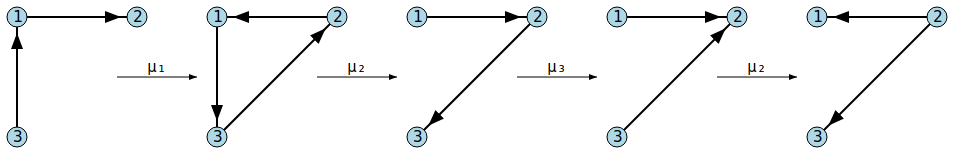The first mutation (μ₁) is on vertex labeled ① and reverses arrows ③→① and ①→②. As a consequence there's a 2-path from ②, via ①, to ③ and we 'complete the tri-cycle' by introducing a new arrow ③→②, thus producing the second quiver in the sequence. Since there were no opposing arrows from ② to ③, it remains.

The second mutation (μ₂) at vertex ② reverses arrows ③→② and ②→①. Consequently there's a 2-path from ① to ③ so we complete ②'s tri-cycle by adding a new arrow from ③ to ①. This time there's already an opposing arrow, ①→③. This would introduce a (forbidden) bi-cycle, so we annihilate the pair.

Note that at this stage (deftly avoiding the word 'point') a reapplication of μ₂ would exactly reverse what we have just done. All quiver mutations performed twice in succession at the same vertex are 'do nothings'.

The remaining mutations on vertices ③ and ② proceed as shown. The final mutation on ② turns that vertex from being a sink (no arrows leave it) into being a source (no arrows enter it).

Note that, in general, mutations on distinct nodes ⓜ and ⓝ do not commute, i.e. a quiver resulting from mutation by μₘ then μₙ is unlikely to be the same as the one resulting from μₙ then μₘ.

For this particular set of three vertices, 14 distinct configurations are possible with various applications of the three mutations: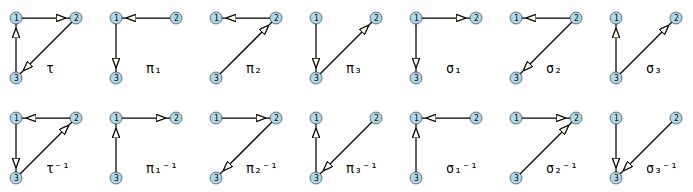In the above, we've labeled the configurations with a τ to suggest a triangle, πₙ to suggest a path through vertex n, and σₙ to suggest a source at vertex n. The inverses, e.g. τ⁻¹ and σₙ⁻¹ (a sink instead of a source) are essentially the same but with all arrows reversed. The following graph (edges bi-directional) shows all 21 ways of mutating between the 14 configurations.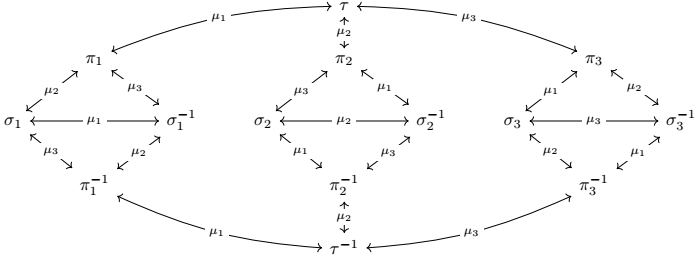The operations μ₁, μ₂ and μ₃ permute τ, π₁, π₂, π₃, σ₁, σ₂, σ₃, τ⁻¹, π₁⁻¹, π₂⁻¹, π₃⁻¹, σ₁⁻¹, σ₂⁻¹, σ₃⁻¹ without affecting the 'shape' of that graph (the vertex labels just - in this case - swap places seven pairs at a time). Thus we have a group which acts upon, and thereby represents the symmetries of, the structure held by these vertices. For example the first generator, μ₁, effects the seven simultaneous transpositions: (π₁,τ)(π₂,σ₂⁻¹)(π₃,σ₃)(σ₁,σ₁⁻¹)(σ₂,π₂⁻¹)(σ₃⁻¹,π₃⁻¹)(τ⁻¹,π₁⁻¹). And mutations μ₂ and μ₃ act - mutatis mutandis - similarly. The group generated is (C₂×C₂×C₂×C₂×C₂×C₂)⋉S₇, the semidirect product of the group (of order 64) formed from the direct product of 6 cyclic groups of order 2 with the group (of order 5040) of permutations of 7 symbols. This quiver group is of order 322560 = 7!(₈C₇)² = 7! × 64 = 2¹⁰×3²×5×7. The order of this group is 'coincidentally' the same as the number of ways 7 rooks may be placed on a standard 8×8 chessboard such that none may attack another.

## Musical Mutations

Needless to say, mutations on quivers with more than three vertices can get considerably more complicated. In general, there will be no limit to the number of configurations. If we consider the six possible mutations possible on the vertices of the above Cantus 1 distillation we can see, for example, that there are four 2-paths through the D vertex, i.e. ADC, ADF, EDC, EDF. All four of these 2-paths will be inverted by a mutation at D and will thereby engender four new arrows AC, AF, EC and EF; the last of these cancelling the existing arrow FE. Thus a quiver with - originally - 8 arrows becomes a quiver with 11. Mutations will always preserve the vertex set but, in general, the number of arrows will typically increase at each application as arrow generation will typically exceed any consequent cancellations.

Although it's possible to have finite quiver groups on six vertices, our trio of Padbrué 'compressings' - all, as it happens, with six vertices - are of infinite order. The six possible 'first mutations', for each quiver, are shown in the following diagram: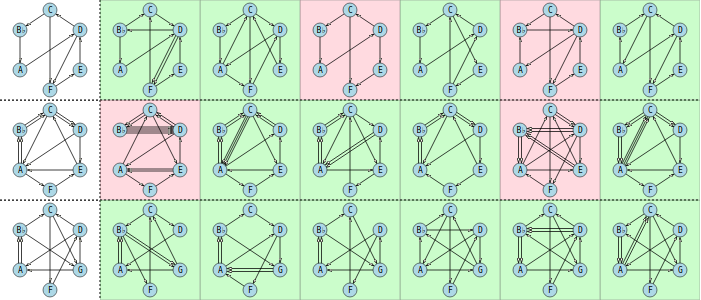The first column (with the uncoloured background) carries the reduction into quivers of each of the three lines in the Pavanne. The remaining six colums show, for each melody, the quivers resulting from mutations on each of the original quiver's six pitches in the order C, D, E, F, A and B♭ for canti 1 and 2 and in the order C, D, F, G, A and B♭ for the bass line. The green backgrounds denote quivers which may be fully traversed, in that it's possible to walk a path from some start vertex to some end vertex consuming each arrow exactly once. The pink backgrounds indicate quivers where this is impossible. This answers - in the negative - the question of its always being possible 'to find at least one path using all arrows in the graph' asked in the previous post.

Clearly a fully traversed path of n arrows will have n + 1 pitches in it from start to finish. Note that such paths are not cycles (Hamiltonian or otherwise) since no 'loop' is completed by terminating at the start node (one may certainly end at the same pitch one began on, but as each pitch may (in general) be visited many times in a traversal, such visits count as separate 'versions').

The following table shows the number of arrows in each of these (first) mutations and also - where possible - the number of full path traversals.

Cantus Iμ(C)μ(D)μ(E)μ(F)μ(A)μ(B♭)
arrows:810107899
# traversals:3614-3-5
Cantus IIμ(C)μ(D)μ(E)μ(F)μ(A)μ(B♭)
arrows:13161513121615
# traversals:130-31513010-315
Bassusμ(C)μ(D)μ(F)μ(G)μ(A)μ(B♭)
arrows:11111110111313
# traversals:5544445121234234

We've already shown above (and in the previous post) some melodies arising from full traversals of the unmutated quivers. We see that it's always possible to find a full traversal after one mutation at D for all lines (e.g. CDACB♭AFDECF for cantus 1, B♭CEDCADCAFEAB♭CAB♭ for cantus 2, and GADFAB♭GAB♭CDG for the bass line. Mutations at F for both canti provide melodies FCEFDCB♭AD and ECDEFAB♭CDAB♭CA. There's no such animal available for the bass - not because there are no possible traversals but because there's no F to mutate on. Instead we can mutate on G and find full traversals, one of which is FDGB♭CAB♭DAGCF. All three lines can provide full traversals after a mutation on B♭, e.g. ADCAB♭CFEDF, DECDACB♭ACB♭AFEACD and B♭ACFDCGDACB♭AGB♭.

## Abstraction to Application

We present a short study using the mutations mentioned. As always, if the player doesn't work, there's some ogg vorbis over here.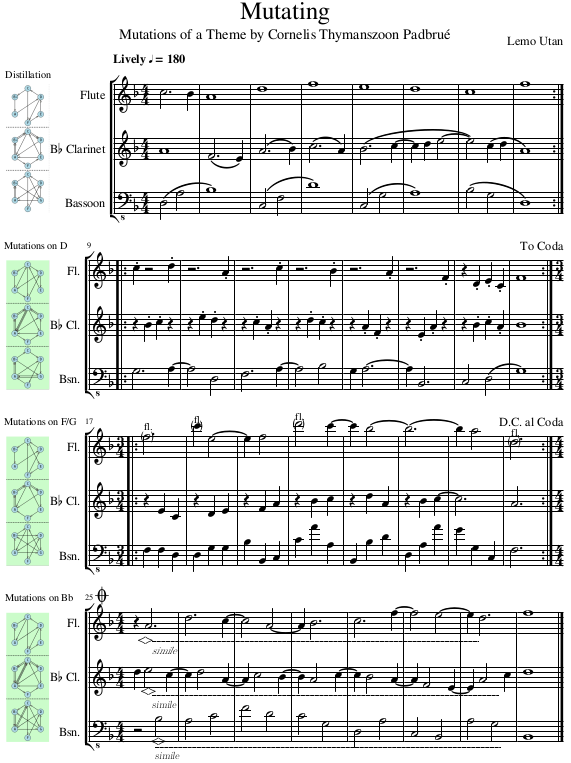Naturally one need not stop at one mutation, nor need one mutate on the same pitch, simultaneously, for each line. As long as one does not mutate a quiver on the same pitch twice (which simply takes you back to where you were) the mutations are - in general - endless (but not for the simple 14 state explanatory model presented earlier). In this particular example, we're always going to be in F major (or D minor, or E Locrian, G Dorian etc), but clearly 'seed' material from more chromatic pieces will tend to stay chromatic.

Note that there's no guarantee that, after each mutation, a full 'all-arrow-consuming' traversal is possible across a quiver one ends up with. But if they do exist then the more often one mutates, the more such possible traversals will exist and the longer will they become. In any case, there's no law forcing you to use only mutations which result in fully traversible quivers.